## Mathematical Surd Operations can be Solved by Nickzom Calculator – The Calculator Encyclopedia

According to Laerd Mathematics,

Surds are numbers left in root form (√) to express its exact value. It has an infinite number of non-recurring decimals. Therefore, surds are irrational numbers.

According to Math is Fun,

• When it is a root and irrational, it is a surd
• Not all roots are surds

Nickzom Calculator solves calculations on surd and these calculations are categorized into:

• Surd Simplification A
• Surd Simplification B
• ‘Binary’ Surd Addition and Subtraction
• ‘Tenary’ Surd Addition and Subtraction
• Surd Multiplication
• Surd Division
• Surd Expansion
• Surd RationalizationNickzom makes use of a certain procedure to help the user easily input the surd values which is clearly represented in the image below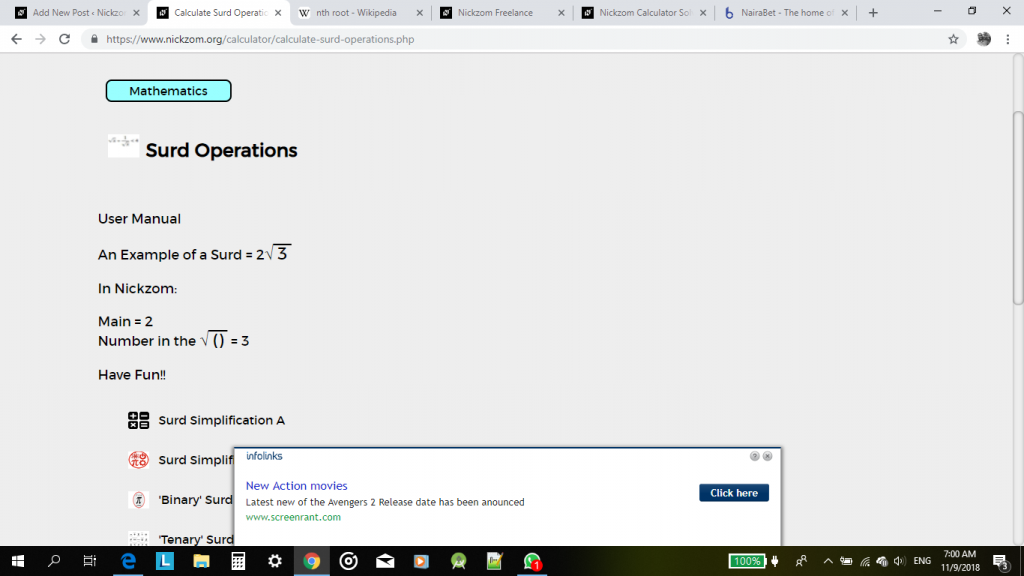For the purpose of this post, we would be handling a step by step guide on how to solve surd rationalization using Nickzom Calculator.

One can access Nickzom Calculator via any of these channels:

Now, click on Surd Rationalization, then enter the values appropriately using the user manual format.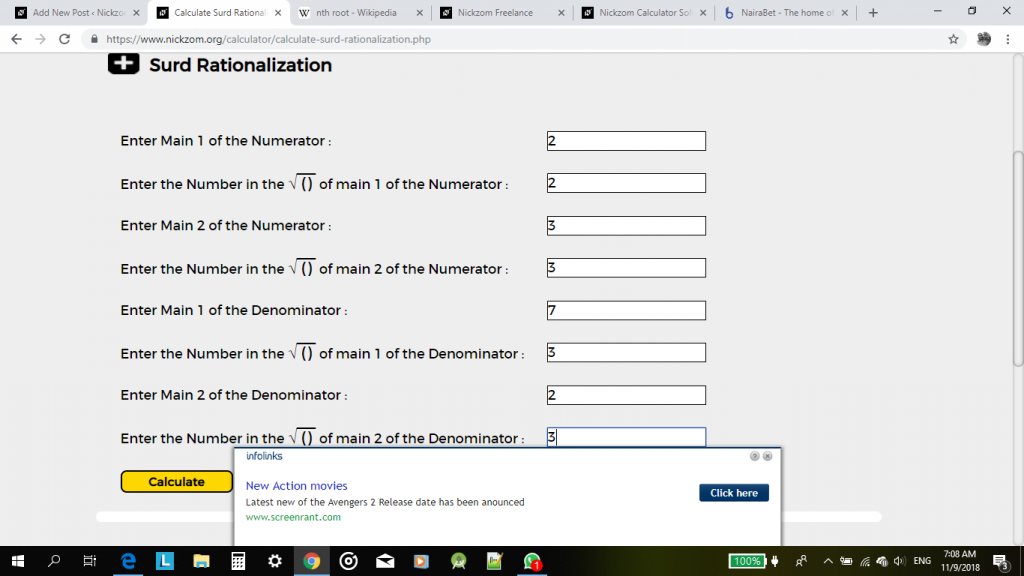Now, click on calculate to display the result.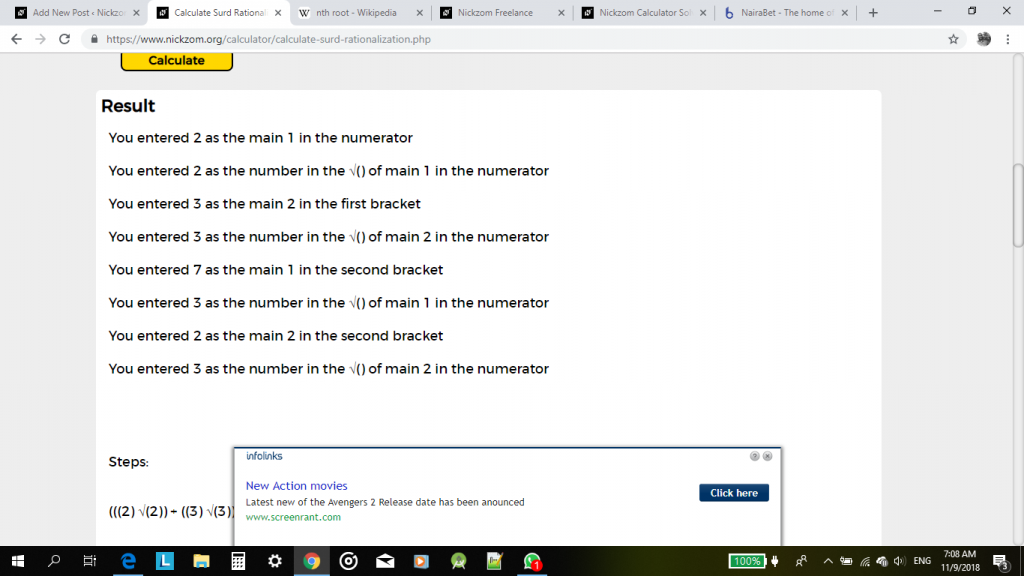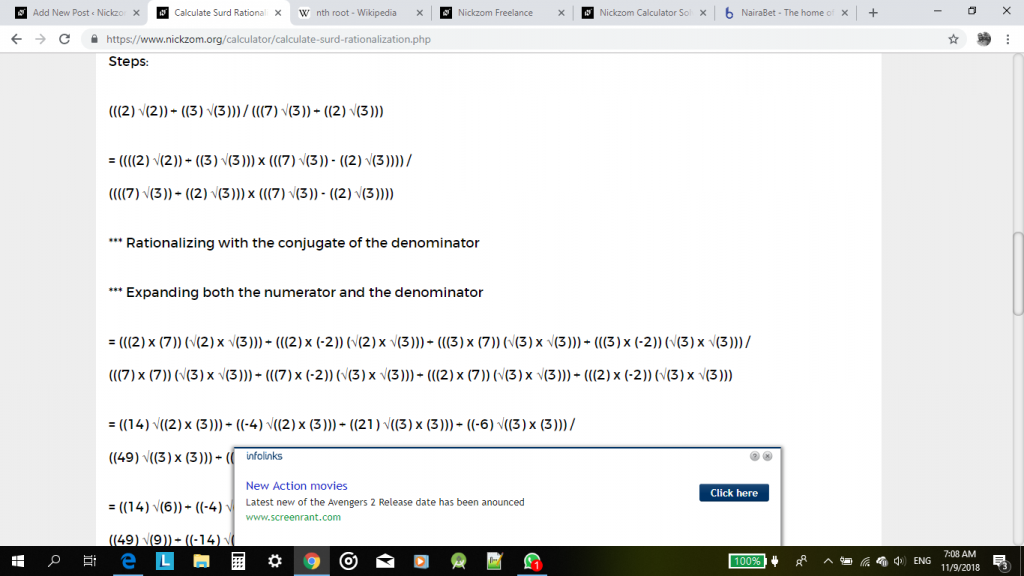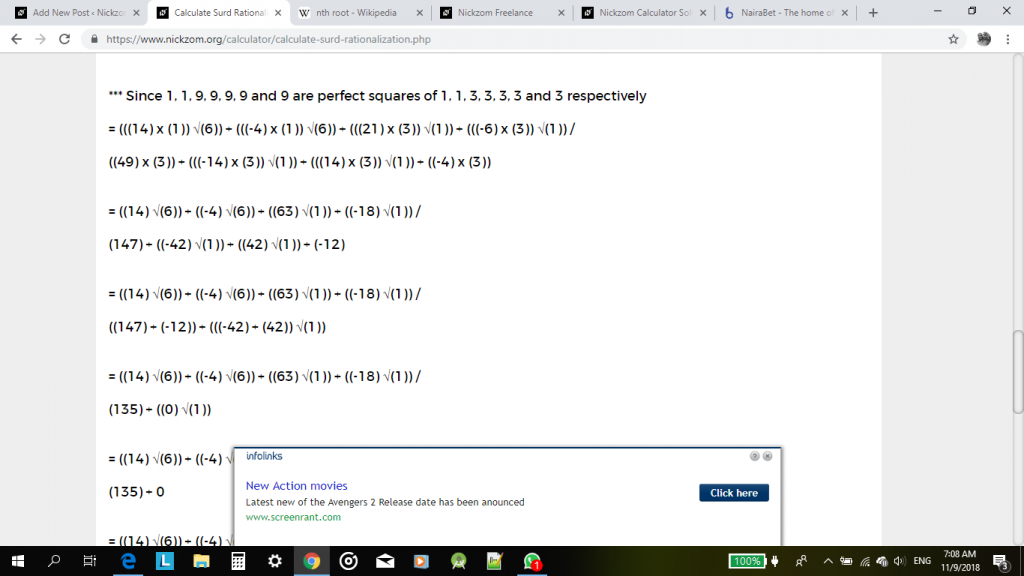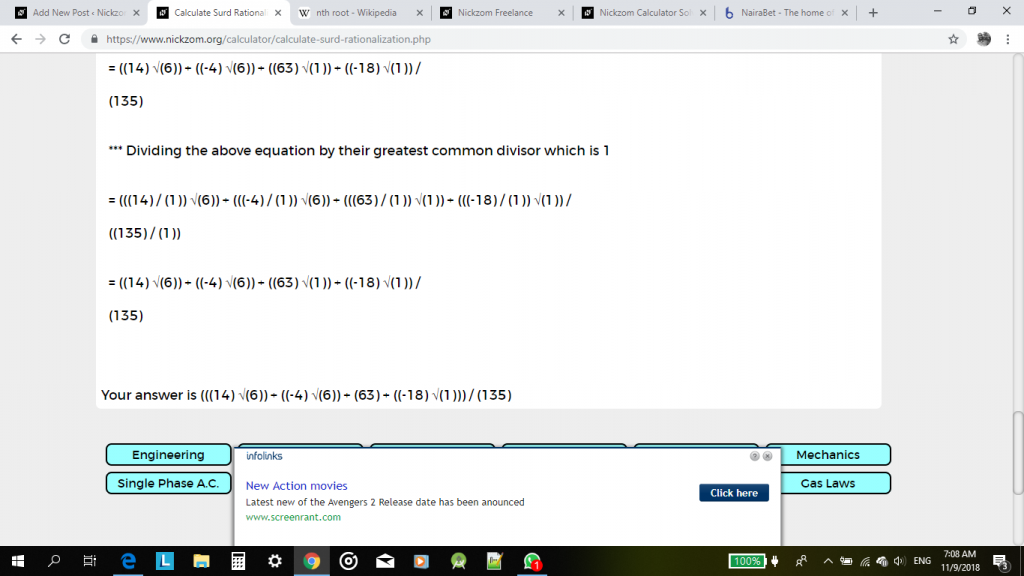I have seen that this steps and procedures might me cumbersome and a bit confusing for a reader or user. Therefore, an update would be coming soon with a new format of solving surd operations that can be easily understood and analysed. For now, with a bit of effort it could be understood or interpreted too.

Watch Out for the Update, Guys!